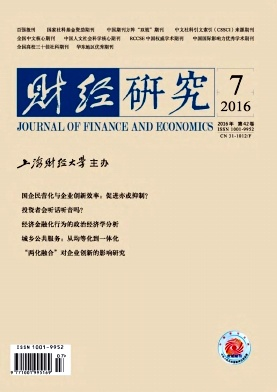﻿ 经济金融化行为的政治经济学分析——一个演化博弈框架
 财经研究2016, Vol. 42Issue (7): 52-62,740

#### 文章信息财经研究2016年42卷第7期

Lu Chunyi, Ding Xiaoqin.

Political Economics Analysis of Economic Financialization Behavior: An Analytical Framework of Evolutionary Game Theory

Journal of Finance and Economics, 2016, 42(7): 52-62,74.

### 文章历史

1.上海立信会计学院 金融学院，上海 201620;
2.复旦大学 经济学院，上海 200433;
3.上海财经大学 马克思主义学院，上海 200433

Political Economics Analysis of Economic Financialization Behavior: An Analytical Framework of Evolutionary Game Theory
Lu Chunyi1,2, Ding Xiaoqin3
1.School of Finance, Shanghai Lixin University of Commerce, Shanghai 201620, China;
2.School of Economics, Fudan University, Shanghai 200433, China;
3.School of Marxism, Shanghai University of Finance and Economics, Shanghai 200433, China
Abstract: Economic financialization has been the focus issue both at home and abroad since the 2008 subprime crisis.From a perspective of political economics,this paper points out the nature of financialization,namely capital accumulation transforms into capital appreciation process out of production and exchanges of surplus value and through financial systems.And then it establishes an asymmetry evolutionary game model with heterogeneity agents to analyze the dynamic evolutionary process of the relationship between non-financial and financial agents from ordinary economic relations to financial relations and its effect mechanism.The results are shown as follows: firstly,the dynamic relation evolution among economic agents indicates that non-financial firms obtain profits mainly through financial practices,financial firms focus on intermediary business and off-balance-sheet business and incorporate ordinary households into their system to make them new profits resources,and ordinary households are forced to accept the two-time benefit sharing from powerful economic agents; the changes in these relations lead to economic financialization and even a financial crisis in a country; secondly,economic development state depends on the interaction of financial and non-financial agents,and the behavior of non-financial agents plays a dominant role; under the given assumptions,when non-financial agents affect predatory technology of financial agents only through their own resources protection,a national economy may be promoted to a new stable state or a meltdown; when non-financial agents affect predatory technology of financial agents through their own resources protection and predatory technology,the economy can enter into an evolutionary stable state;thirdly,the impacts of financialization behavior of economic agents can be divided into three dimensions: financialization behavior of economic agents promote their own excess capital accumulation in the short run,the predatory behavior of financial agents and the production behavior of non-financial agents always present an opposite economic relation which is easily intensified,and free market without government intervention inevitably leads to the intensification of conflicts and falls into crises.Therefore,deep understanding of the nature of economic finacialization and its mechanism of affecting the economy is of great significance to both economic transformation and financial reform in China.
Key words: finacialization    evolutionary game theory    political economics    predatory technology    evolutionary stable strategy

20世纪中后叶，资本主义国家开始推行新自由主义，从以往通过政府权力投入货币流量来实现扩大再生产的模式，转变为以金融自由化为特征的市场来创造货币流量支撑扩大再生产。于是，资本家热衷于通过创造金融产品以分割剩余价值，而不再通过生产商品、创造剩余价值来获得利润，从而出现了金融繁荣与生产萎缩的经济状况，最终导致了2008年蔓延多国的金融危机。然而，有关经济金融化的本质、影响乃至生成机制等方面的研究仍存在争议，尤其是西方经济学和马克思主义经济学的相关研究颇有不同。

(二) 演化博弈在金融化分析中的框架设定

 $dx/dt=x({{u}_{1}}-\bar{u})=x\left( 1-x \right)(\text{ }{{u}_{1}}-\text{ }{{u}_{2}})$ (1)

1. 经济中有两类经济主体(非金融主体和金融主体)。非金融主体可选择的策略为(参与投机，专注生产)，金融主体可选择的策略为(从事投机，支持生产)。投机行为是对生产行为的一种分利，即分割生产的剩余价值。

2. 在给定的经济环境中，非金融主体参与投机但并不会转变为金融主体，金融主体可支持生产但并不会转变为非金融主体。

3. 双方的策略过程基于有限理性的假设进行，且每个主体可根据其他成员的策略选择考虑其在自身群体中的相对适应性来选择和调整各自的策略。

4. 参与投机的非金融主体占比为x，则专注于生产的非金融主体比重为1-x，定义大量非金融主体专注生产的社会为生产型社会，反之为投机型社会；从事投机活动的金融主体的比重为y，而支持生产的金融主体的比重为1-y。从事金融化分利 支持生产 参与金融化分利 ＞Aθ1，Aθ2 Aθ1，R 专注生产 αA(1- θ2+f)，αA(θ2-f) βA，βR

 ${{U}_{11}}=yA{{\theta }_{1}}+\left( 1-y \right)A{{\theta }_{1}}$ (2)

 ${{U}_{12}}=y\alpha A(1-{{\theta }_{2}}+f)+\left( 1-y \right)\text{ }\beta A$ (3)

 ${{U}_{1}}=x{{U}_{11}}+\left( 1-x \right){{U}_{12}}$ (4)

 \begin{align} &F\left( X \right)=dx/dt=x({{U}_{11}}-\text{ }{{U}_{1}})=x\left( 1-x \right)({{U}_{11}}-\text{ }{{U}_{12}}) \\ &=Ax\left( 1-x \right)\{(\text{ }{{\theta }_{1}}+\beta )-y[\alpha \text{ }(1-\text{ }{{\theta }_{2}}+f)+\text{ }\beta]\} \\ \end{align} (5)

 ${{U}_{21}}=xA{{\theta }_{2}}+\left( 1-x \right)\alpha A({{\theta }_{2}}-f)$ (6)

 ${{U}_{22}}=xR+\left( 1-x \right)\beta R$ (7)

 ${{U}_{2}}=y{{U}_{21}}+\left( 1-y \right){{U}_{22}}$ (8)

 \begin{align} &F\left( y \right)=dy/dt=y({{U}_{21}}-\text{ }{{U}_{2}})=y\left( 1-y \right)({{U}_{21}}-\text{ }{{U}_{22}}) \\ &=y\left( 1-y \right)\{x[{{\theta }_{2}}A-R+\text{ }\beta R-\text{ }\alpha A(\text{ }{{\theta }_{2}}-f)\left] - \right[\beta R-\alpha A(\text{ }{{\theta }_{2}}-f)]\} \\ \end{align} (9)
(二) 经济主体的金融化行为演化稳定分析

1.非金融主体的金融化行为演化稳定分析。根据演化稳定策略理论，F(x)=0时可以解得有可能出现的平衡点，即x*=0(非金融主体都专注生产)和x*=1(非金融主体都参与投机)是两个可能的稳定状态点。非金融主体的演化行为选择取决于y，其中y*为分界点，且y*=(θ1+β)/[α(1-θ2+f)+β]。令μ=α(1-θ2+f)，则y*=(θ1+β)/(μ+β)=1-(μ-θ1)/(μ+β)。下面根据几个极端点分析非金融主体的金融化行为演化动态。

2.金融主体的金融化行为演化稳定分析。同样根据演化稳定策略理论，F(y)=0时可以解得有可能出现的平衡点，即y*=0(金融主体都支持生产)和y*=1(金融主体都从事投机活动)是两个可能的稳定状态点。金融主体的演化行为选择取决于x，其中x*为分界点，且x*=[βR-αA(θ2-f)]/[Aθ2-R+βR-αA(θ2-f)]。令ω=βR-αA(θ2-f)，则x*=ω/[ω-(R-Aθ2)]。

(三) 经济主体金融化行为动态复制系统的稳定性分析

 $dx/dt=\text{ }Ax\left( 1-x \right)\{(\text{ }{{\theta }_{1}}+\beta )-y[\alpha \text{ }(1-\text{ }{{\theta }_{2}}+f)+\text{ }\beta]\}$ (10)
 $dy/dt=\text{ }y\left( 1-y \right)\{x[{{\theta }_{2}}A-R+\text{ }\beta R-\text{ }\alpha A(\text{ }{{\theta }_{2}}-f)\left] - \right[\beta R-\alpha A(\text{ }{{\theta }_{2}}-f)]\}$ (11)

 \eqalign{ &J = \left| {{{\partial F\left( x \right)} \over {\partial x}}{{\partial F\left( x \right)} \over {\partial y}}{{\partial F\left( y \right)} \over {\partial y}}{{\partial F\left( y \right)} \over {\partial x}}} \right| = \cr &\left| \matrix{ A\left( {1 - 2x} \right)({\theta _1} + \beta ) - y\alpha (1 - {\theta _2} + f) + \left. \beta \right\} - Ax\left( {1 - x} \right)\left[ {\alpha (1 - {\theta _2} + f) + \beta } \right] \hfill \cr y\left( {1 - y} \right)\left[ {{\theta _2}A - R + \beta R - \alpha A({\theta _2} - f)} \right]\left( {1 - 2y} \right)x({\theta _2}A - R) - \left( {1 - x} \right)\left. {\left[ {\beta R - \alpha A({\theta _2} - f)} \right]} \right\} \hfill \cr} \right| \cr}

 $Det\left( J \right)=\left[\partial F\left( x \right)/\partial x \right]\left[\partial F\left( y \right)/\partial y \right]-\left[\partial F\left( x \right)/\partial y \right]\left[\partial F\left( y \right)/\partial x \right]$ (12)
 $Tr\left( J \right)=\partial F\left( x \right)/\partial x+\partial F\left( y \right)/\partial y$ (13)

 局部均衡点 De(J)的符号 Tr(J)的符号 稳定性 条 件 E1(1，0) + - ESS 0 ＜θ2 ＜R(1-β)/[A(1-α)]+αf/(1-α) - +/- 鞍点 其他条件 E2(0，0) + + 不稳定 任意条件 E3(0，1) + - ESS βR/(αA)+f ＜θ2 ＜(α-θ1)/α+f - +/- 鞍点 其他条件 E4(1，1) + - ESS (α-θ1)/α+f ＜θ2 ＜1 - +/- 鞍点 其他条件 E5 +/- 0 鞍点 任意条件

(1)当金融主体的分利技术系数(θ2)在区间1时，E1(1，0)为演化稳定状态。也就是说，在经济主体为保护生产所支付的资源(f)给定的前提下，此时社会中非金融主体和金融主体最终会形成(参与金融化分利，支持生产)的稳定状态；而当经济主体为保护生产所支付的资源(f)增加时，为保持稳定状态，θ2自然也会提高。如果金融主体的分利技术(θ2)给定，那么经济主体为保护生产所支付的资源(f)将会小于R(1-β)/(αA)+(α-1)θ2/α。显然，如果θ2提高，为保持稳定状态，f自然也会提高。由此可以看到，金融主体的分利技术和非金融主体为保护生产所支付的资源决定了经济中最后的稳定状态。

(2) 当金融主体的分利技术系数(θ2)在区间2时，上述各局部均衡点都不是演化稳定点，所以此时没有演化稳定策略。也就是说，如果金融化主体的分利技术(θ2)在这个范围里，社会不会形成演化稳定策略。此时非金融主体和金融主体可能选择(专注生产，支持生产)的策略，也可能选择其他策略，但不会形成持久的稳定状态。

(3) 当金融主体的分利技术系数(θ2)在区间3时，E3(0，1)是演化稳定策略。也就是说，在经济主体为保护生产所支付的资源(f)给定的前提下，此时社会中非金融主体和金融主体最终会形成(专注生产，从事金融化分利)的稳定状态。而当经济主体为保护生产所支付的资源(f)增加时，为保持稳定状态，θ2自然也会提高；当非金融主体的分利技术(θ1)提高时，为保持稳定状态，θ2就会降低。如果金融主体的分利技术(θ2)给定，那么经济主体为保护生产所支付的资源(f)在((αθ2-α+θ1)/α，(αAθ2-βR)/(αA))范围内。因此，无论是θ2θ1提高，为保持稳定状态，f都会提高。

(4) 当金融主体的分利技术系数(θ2)在区间4时，E4(1，1)为演化稳定状态。也就是说，在经济主体为保护生产所支付的资源(f)给定的前提下，此时社会中非金融主体和金融主体最终会形成(参与金融化分利，从事金融化分利)的稳定状态。而当经济主体为保护生产所支付的资源(f)增加时，为保持稳定状态，θ2自然也会提高；当非金融主体的分利技术(θ1)提高时，为保持稳定状态，θ2就会降低。如果金融主体的分利技术θ2给定，那么经济主体为保护生产所支付的资源(f)将大于(αθ2-α+θ1)/α。因此，无论是θ2θ1提高，为保持稳定状态，f都会提高。

(二) 金融企业的金融化行为方式及其经济关系

(三) 普通家庭被动金融化行为及其与其他主体的关系

(四) 经济主体金融化行为的现实影响

  考斯达斯·拉帕维查斯, 李安. 金融化了的资本主义:危机和金融掠夺[J].政治经济学评论,2009(1):30–58.  鲁春义. 垄断、金融化与中国行业收入分配差距[J].管理评论,2014(11):48–56.  马克思. 资本论[M]. 北京: 人民出版社, 2004 .  马慎萧. 资本主义"金融化转型"是如何发生的?——解释金融化转型机制的四种研究视角[J].教学与研究,2016(3):71–79.  [德]希法亭.金融资本[M].福民等译.北京:商务印书馆,1994.  肖斌.金融化进程中的资本主义经济运行透视[D].成都:西南财经大学,2013.  Hunt E K. Marx as a social economist: The labor theory of value[J].Review of Social Economy,1979,37(3): 275–294.  Jeanneney S G,Kpodar K.Financial development,financial instability and poverty[R].CSAE Working Paper No.2005-09,2011.  Krippner G R. Capitalizing on crisis: The political origins of the rise of finance[M]. Cambridge,MA: Harvard University Press, 2011 .  Lapavitsas C. Theorizing financialization[J].Work,Employment&Society,2011,25(4): 611–626.  Lazonick W, O'Sullivan M. Maximizing shareholder value: A new ideology for corporate governance[J].Economy and Society,2000,29(1): 13–35.  Levine R,Rubinstein Y.Liberty for more: Finance and educational opportunities[R].NBER Working Paper No.19380,20l3.  Palley T.Financialization: What is it and why it matters[R].Political Economy Research Institute Working Paper No.153,2007.